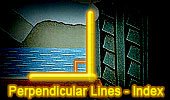Perpendicular Lines, Theorems and Problems - Table of Content  (14)Geometry Problem 635 Semicircle, Diameter, Perpendicular, Inscribed Circle, Radius. Geometry Problem 629 Triangle, Perpendicular to Sides, Intersecting Lines, Altitude. Geometry Problem 613 Altitude of a Triangle, Perpendicular, Collinear Points. Geometry Problem 612 Altitude of a Triangle, Perpendicular, Congruence. Geometry Problem 611 Altitude of a Triangle, Perpendicular, Collinear Points, Parallel. Geometry Problem 610 Intersecting Circles, Radius, Parallel, Common Tangent, Angle, 90 Degrees. Geometry Problem 609 Triangle, Perpendiculars, 90 Degrees, Collinearity. Problem 606 Isosceles Triangles, Perpendicular and Parallel Lines. Geometry Problem 589. Triangle, Incenter, Incircle, Tangency Points, Midpoint, Perpendicular, Distance. Problem 578. Circle, Perpendicular Chords, Midpoints, Curvilinear Triangle, Area, 90 Degrees. Proposed Problem 437. Tangent Circles, Diameter, Chord, Perpendicular, Congruence, Measurement. Proposed Problem 436. Tangent Circles, Diameter, Chord, Perpendicular, Congruence, Measurement. Proposed Problem 430. Circumscribed and Inscribed Regular Pentagon, Perpendicular, Areas. Proposed Problem 429. Circumscribed and Inscribed Regular Pentagon, Perpendicular, Measurement. Proposed Problem 428. Quadrant of a circle, Square, Angle bisector, Measurement. Go to Page: Previous | 1 | 2 | 3 | 4 | 5 | 6 | 7 | 8 | 9 | 10 | 11 | 12 | 13 | 14 | 15 | 16 | 17 | 18 | 19 | 20 | Next

 Home | Search | Geometry | Post a comment | Email | Post a comment | By Antonio Gutierrez Last updated: Feb 15, 2015